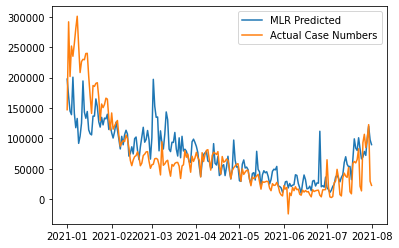# Using Linear Layer and Manually Implementing Linear Layer gives different results

So pretty much what the title says. Using nn.Linear and manually implementing it keeps giving me different results. The loss for nn.Linear is much higher than the loss I see when I manually implement it (to the point where results from nn.Linear is unusable). Here is the code that I have.

Manual Implementation:

``````num_cols = 3  # Postive, Negative, Neutral

def model(X):
return A.mm(x_data) + b

def loss(y_predicted, y_target):

num_epochs = 10000

with trange(num_epochs, desc="Training Multi-variable Linear Regression") as progress_bar:
for _ in progress_bar:
y_pred = model(x_data)
curr_loss = loss(y_pred, y_data)
curr_loss.backward()
optimizer.step()
progress_bar.set_postfix(loss=curr_loss.data)
# print(f"Epoch: {curr_epoch}, Loss: {curr_loss}")
``````

Graph Produced:Weights after training: `tensor([[ 165.0836, 307.2172, -130.3902]], requires_grad=True)`

PyTorch’s Linear Layer:

``````class PlainSentimentCaster(nn.Module):
def __init__(self, DIM_FEATURES=3):
super().__init__()
self.DIM_FEATURES = DIM_FEATURES
self.lin_layer1 = nn.Linear(self.DIM_FEATURES, 1)

def forward(self, X):
return self.lin_layer1(X)

def train(model: nn.Module, X, Y_target, num_epochs=10000):
with trange(num_epochs, desc="Training TF-IDF Sentiment Linear Layer Model") as progress_bar:
for _ in progress_bar:
y_pred = model.forward(X)
curr_loss = loss(y_pred, Y_target)
curr_loss.backward()
optimizer.step()
progress_bar.set_postfix(loss=curr_loss.data)

baseline_model_3 = PlainSentimentCaster(DIM_FEATURES=3)
train(baseline_model_3, x_data.float().t(), y_data.float().t())
``````

Graph Produced:
https://imgur.com/a/WKtgzUU
Weights after training: `tensor([[-0.9212, -1.6551, 2.8089]], requires_grad=True)`

Does anyone know what might be going on here?

Firstly, I’d check you use the same starting weights by printing the weights at the beginning of training and fix the RNG seed via `torch.manual_seed`. Also, `nn.Linear` uses kaiming_uniform initialisation where you’re using `torch.randn` which is a distributed in accordance to a gaussian. So, your initial weights are most likely entirely different.

Secondly, your manual implementation of the Linear layer differs from the `nn.Linear` (Linear — PyTorch 1.10.0 documentation).

Your implementation is y = A@x + b whereas PyTorch’s implemenetation is x@A^T + b (where A and b are the weight and bias respectively). Although the output shape is the same, the derivatives are different w.r.t A, e.g. dy/dA is x is the first case and x^T in the second case.